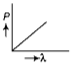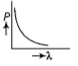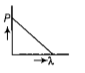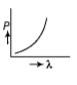The maximum kinetic energy of photoelectron emitted from the surface of work function f due to incidence of light of frequency n is E. If the frequency of incident light is doubled, then maximum kinetic of emitted photon will be

1.  2E

2.  2E - f

3.  2E + f

4.  2E + 2f

Subtopic:  Einstein's Photoelectric Equation |
To view explanation, please enrol in the course below.
NEET 2021 - Achiever Batch - Aryan Raj Singh

Difficulty Level:

The de-Broglie associated with an electron accelerated through a voltage of 900 V is:

1.

2.

3.

4.

Subtopic:  De-broglie Wavelength |
To view explanation, please enrol in the course below.
NEET 2021 - Achiever Batch - Aryan Raj Singh

Difficulty Level:

The de-Broglie wavelength of a neutron in thermal equilibrium with heavy water at a temperature T (Kelvin) and mass m, is

(1)$\frac{h}{\sqrt{mkT}}$

(2)$\frac{h}{\sqrt{3mkT}}$

(3)

(4)$\frac{2h}{\sqrt{mkT}}$

Subtopic:  De-broglie Wavelength |
To view explanation, please enrol in the course below.
NEET 2021 - Achiever Batch - Aryan Raj Singh

Difficulty Level:

Electrons of mass m with de-Broglie wavelength $\lambda$ fall on the target in an X-ray tube. The cut off wavelength $\left({\lambda }_{0}\right)$ of the emitted X-ray is -

(1) ${\lambda }_{0}=\frac{2mc{\lambda }^{2}}{h}$

(2) ${\lambda }_{0}=\frac{2h}{mc}$

(3) ${\lambda }_{0}=\frac{2{m}^{2}{c}^{2}{\lambda }^{3}}{{h}^{2}}$

(4) ${\lambda }_{0}=\lambda$

Subtopic:  Davisson & Germer Experiment |
To view explanation, please enrol in the course below.
NEET 2021 - Achiever Batch - Aryan Raj Singh

Difficulty Level:

Photons with energy 5 eV are incident on a cathode C in a photoelectric cell. The maximum energy of emitted photoelectrons is 2 eV. When photons of energy 6 eV are incident on C, no photo electron will reach the anode A, if the stopping potential of A relative to C is

1. +3 V             2. +4 V

3. - 1V              4. -3 V

Subtopic:  Einstein's Photoelectric Equation |
To view explanation, please enrol in the course below.
NEET 2021 - Achiever Batch - Aryan Raj Singh

Difficulty Level:

When a metallic surface is illuminated with radiation of wavelength $\lambda$, the stopping potential is V. If the same surface is illuminated with radiation of wavelength 2$\lambda$, the stopping potential is $\frac{V}{4}$ .The threshold wavelength for metallic surface is:

(a) 5$\lambda$                (b)$\frac{5}{2}$$\lambda$

(c) 3$\lambda$                (d) 4$\lambda$

Subtopic:  Photoelectric Effect: Experiment |
To view explanation, please enrol in the course below.
NEET 2021 - Achiever Batch - Aryan Raj Singh

Difficulty Level:

An electron of mass m and a photon have the same energy E. Find the ratio of de-Broglie wavelength associated with the electron to that associated with the photon. (c is the velocity of light)

Subtopic:  De-broglie Wavelength |
To view explanation, please enrol in the course below.
NEET 2021 - Achiever Batch - Aryan Raj Singh

Difficulty Level:

A radiation of energy 'E' falls normally on a perfectly reflecting surface. The momentum transferred to the surface is (c=velocity of light)

1. E/c

2. 2E/c

3. 2E/c2

4. E/c2

Subtopic:  Particle Nature of Light |
To view explanation, please enrol in the course below.
NEET 2021 - Achiever Batch - Aryan Raj Singh

Difficulty Level:

A certain metallic surface is illuminated with monochromatic light of wavelength λ. The stopping potential for photoelectric current for this light is 3Vo. If the same surface is illuminated with light of wavelength 2λ. the stopping potential is Vo. The threshold wavelength for this surface for the photoelectric effect is:

1. 6λ

2. 4λ

3. λ/4

4. λ/6

Subtopic:  Einstein's Photoelectric Equation |
To view explanation, please enrol in the course below.
NEET 2021 - Achiever Batch - Aryan Raj Singh

Difficulty Level:

Which of the following figures represent the variation of particle

momentum and the associated de-Broglie wavelength?

1.2.3.4.Subtopic:  De-broglie Wavelength |
To view explanation, please enrol in the course below.
NEET 2021 - Achiever Batch - Aryan Raj Singh

Difficulty Level: